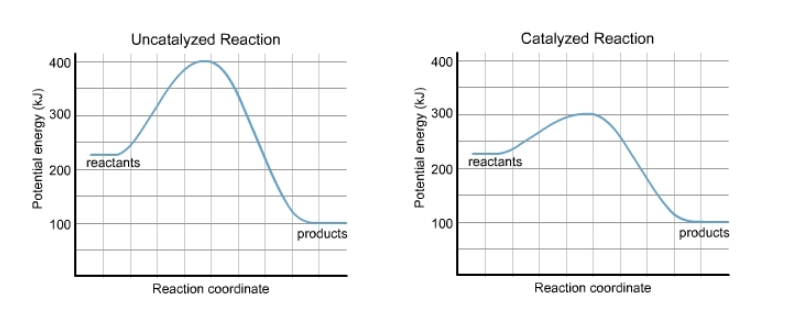Problem: Please refer to the charts. a) What is the value of the activation energy of the uncatalyzed reaction? activation energy = ____________ b) What is the value of the enthalpy change of the uncatalyzed reaction? ΔH = ____________ c) What is the value of the activation energy of the uncatalyzed reaction in reverse? activation energy = ____________ d) What is the value of the enthalpy change of the uncatalyzed reaction in reverse? ΔH = ____________

FREE Expert Solution
80% (253 ratings)
Problem Details

a) What is the value of the activation energy of the uncatalyzed reaction?

activation energy = ____________

b) What is the value of the enthalpy change of the uncatalyzed reaction?

ΔH = ____________

c) What is the value of the activation energy of the uncatalyzed reaction in reverse?

activation energy = ____________

d) What is the value of the enthalpy change of the uncatalyzed reaction in reverse?

ΔH = ____________